rfbudget

Create RF budget object and compute RF budget results for chain of 2-port elements

Description

Use the rfbudget object to create an rfbudget element to calculate RF budget results of a circuit. You can use any 2-port element in this circuit such as amplifier, nport, or modulator. Open the complete rfbudget circuit in an RF Budget Analyzer app. You can also export the completed circuit to RF Blockset™.

Creation

Description

example

rfobj = rfbudget creates an rfbudget object, rfobj, with default empty property values.

example

rfobj = rfbudget(elements,inputfreq,inputpwr,bandwidth) creates an RF budget object from the input RF elements, and independently computes an RF budget analysis at the specified input frequencies, available input power, and signal bandwidth. The input arguments are stored in the Elements, InputFrequency, AvailableInputPower, and SignalBandwidth properties. The analysis results are stored in dependent properties. By default, if any of the input properties are changed, the object recomputes results.

rfobj = rfbudget(___,autoupdate)sets the AutoUpdate property. Setting AutoUpdate to false turns off automatic budget recomputation as parameters are changed. You can use this syntax with any of the previous syntaxes.

rfobj = rfbudget(...,Name,Value)creates RF budget object with additional properties specified by one or more name-value pair arguments. Name is the property name and Value is the corresponding value. You can specify several name-value pair arguments in any order as Name1, Value1, ..., NameN, ValueN. Properties not specified retain their default values.

Properties

expand all

RF budget elements, specified as the comma-separated pair consisting of 'Elements' and an RF toolbox object or array of RF toolbox objects. The possible elements are amplifier, modulator, generic rfelement, and nport objects. To specify a circuit consisting of multiple RF objects, specify the elements as a cell array. For information on edge cases, see Algorithms.

Example: a = amplifier;m = modulator;rfbudget('Elements',[a m]) calculates the RF budget analysis of the amplifier and modulator circuit.

Input frequency of signal, specified as the comma-separated pair consisting of 'InputFrequency' and a scalar or vector in Hz. If the input frequency is a vector, then the RF budget object calculates the analysis for each input frequency separately.

Example: 'InputFrequency',2e9

Data Types: double

Power applied at the input of the cascade, specified as the comma-separated pair consisting of 'AvailableInputPower' and a scalar in dBm.

Example: 'AvailableInputPower',-30

Data Types: double

Signal bandwidth at the input of the cascade, specified as the comma-separated pair consisting of 'SignalBandwidth' and a scalar in Hz.

Example: 'SignalBandwidth',10

Data Types: double

Option to automatically recompute the RF budget analysis by incorporating changes made to the existing circuit, specified as the comma-separated pair consisting of 'AutoUpdate' and a boolean scalar.

Example: 'AutoUpdate',true

Data Types: logical

Computation method, specified as Friis or HarmonicBalance. The Friis solver is faster than the HarmonicBalance solver, but does not support computation of nonlinearities such as OIP2. The HarmonicBalance solver, the tone and harmonic-dependent properties are displayed.

Note

HarmonicBalance does not support architectures where the input or output frequencies at any stage are nonzero and less than SignalBandwidth.

Example: 'Solver','Friis'

Data Types: string

Output frequencies, specified as the comma-separated pair consisting of 'OutputFrequency' and a row vector in Hz.

Data Types: double

Output power, specified as the comma-separated pair consisting of 'OutputPower' and a row vector in dBm.

Data Types: double

Transducer power gains, specified as the comma-separated pair consisting of 'TransducerGain' and a row vector in dB.

Data Types: double

Noise figures, specified as the comma-separated pair consisting of 'NF' and a row vector in dB.

Data Types: double

Input-referred second-order intercept, specified as the comma-separated pair consisting of 'IIP2' and a row vector in dBm. These values are computed only when the selected solver is HarmonicBalance solver.

Data Types: double

Output-referred second-order intercept, specified as the comma-separated pair consisting of 'OIP2' and a row vector in dBm. These values are computed only when the selected solver is HarmonicBalance solver.

Data Types: double

The Output-referred third-order intercept, specified as the comma-separated pair consisting of 'OIP3' and a row vector in dBm.

Data Types: double

The Input-referred third-order intercept, specified as the comma-separated pair consisting of 'IIP3' and a row vector in dBm.

Data Types: double

Signal-to-noise ratio, specified as the comma-separated pair consisting of 'SNR' and a row vector in dB.

Data Types: double

Displays progress bar with cancel button during harmonic balance analysis, specified as true or false.

Data Types: logical

Object Functions

 show Display RF budget object in RF Budget Analyzer app computeBudget Compute results of rfbudget object exportScript Export MATLAB code that generates RF budget object exportRFBlockset Create RF Blockset model from RF budget object exportTestbench Create measurement testbench from RF budget object rfplot Plot cumulative RF budget result versus cascade input frequency smithplot Plot impedance transformation for selected matching network on smith chart polar Plot specified object parameters on polar coordinates

Examples

collapse all

Open a default RF budget object.

obj = rfbudget
obj =
rfbudget with properties:

Elements: []
InputFrequency: [] Hz
AvailableInputPower: [] dBm
SignalBandwidth: [] Hz
Solver: Friis
AutoUpdate: true

Create an amplifier with a gain of 4 dB.

a = amplifier('Gain',4);

Create a modulator with an OIP3 of 13 dBm.

m = modulator('OIP3',13);

Create an nport using passive.s2p.

n = nport('passive.s2p');

Create an rf element with a gain of 10 dB.

r = rfelement('Gain',10);

Calculate the rf budget of a series of rf elements at an input frequency of 2.1 GHz, an available input power of -30 dBm, and a bandwidth of 10 MHz.

b = rfbudget([a m r n],2.1e9,-30,10e6)
b =
rfbudget with properties:

Elements: [1x4 rf.internal.rfbudget.Element]
InputFrequency: 2.1 GHz
AvailableInputPower: -30 dBm
SignalBandwidth:  10 MHz
Solver: Friis
AutoUpdate: true

Analysis Results
OutputFrequency: (GHz) [  2.1    3.1    3.1     3.1]
OutputPower: (dBm) [  -26    -26    -16   -20.6]
TransducerGain: (dB)  [    4      4     14     9.4]
NF: (dB)  [    0      0      0  0.1392]
IIP2: (dBm) []
OIP2: (dBm) []
IIP3: (dBm) [  Inf      9      9       9]
OIP3: (dBm) [  Inf     13     23    18.4]
SNR: (dB)  [73.98  73.98  73.98   73.84]

Show the analysis in the RF Budget Analyzer app.

show(b)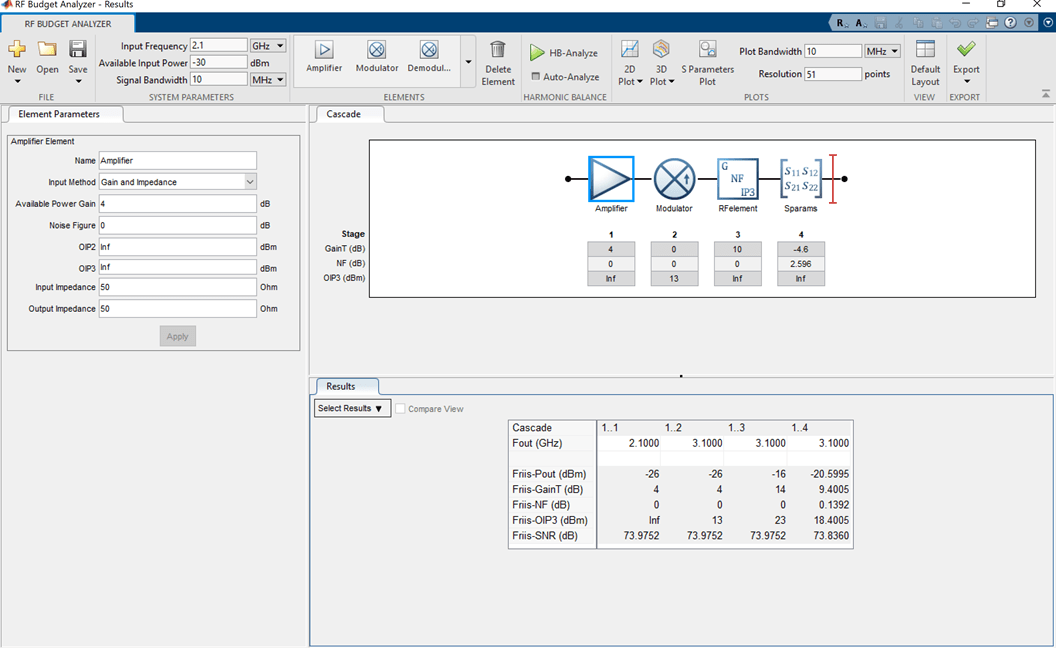Create an RF system.

Create an RF bandpass filter using the Touchstone® file RFBudget_RF.

f1 = nport('RFBudget_RF.s2p','RFBandpassFilter');

Create an amplifier with a gain of 11.53 dB, a noise figure (NF) of 1.53 dB, and an output third-order intercept (OIP3) of 35 dBm.

a1 = amplifier('Name','RFAmplifier','Gain',11.53,'NF',1.53,'OIP3',35);

Create a demodulator with a gain of -6 dB, a NF of 4 dB, and an OIP3 of 50 dBm.

d = modulator('Name','Demodulator','Gain',-6,'NF',4,'OIP3',50, ...
'LO',2.03e9,'ConverterType','Down');

Create an IF bandpass filter using the Touchstone file RFBudget_IF.

f2 = nport('RFBudget_IF.s2p','IFBandpassFilter');

Create an amplifier with a gain of 30 dB, a NF of 8 dB, and an OIP3 of 37 dBm.

a2 = amplifier('Name','IFAmplifier','Gain',30,'NF',8,'OIP3',37);

Calculate the RF budget of the system using an input frequency of 2.1 GHz, an input power of -30 dBm, and a bandwidth of 45 MHz.

b = rfbudget([f1 a1 d f2 a2],2.1e9,-30,45e6)
b =
rfbudget with properties:

Elements: [1x5 rf.internal.rfbudget.Element]
InputFrequency: 2.1 GHz
AvailableInputPower: -30 dBm
SignalBandwidth:  45 MHz
Solver: Friis
AutoUpdate: true

Analysis Results
OutputFrequency: (GHz) [   2.1    2.1   0.07    0.07   0.07]
OutputPower: (dBm) [-31.53    -20    -26  -27.15  2.847]
TransducerGain: (dB)  [-1.534  9.996  3.996   2.847  32.85]
NF: (dB)  [ 1.533  3.064  3.377   3.611  7.036]
IIP2: (dBm) []
OIP2: (dBm) []
IIP3: (dBm) [   Inf     25  24.97   24.97  4.116]
OIP3: (dBm) [   Inf     35  28.97   27.82  36.96]
SNR: (dB)  [ 65.91  64.38  64.07   63.83  60.41]

Plot the available output power.

rfplot(b,'Pout')
view(90,0)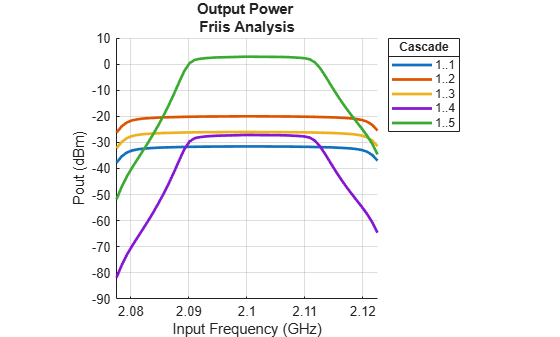Plot the transducer gain.

rfplot(b,'GainT')
view(90,0)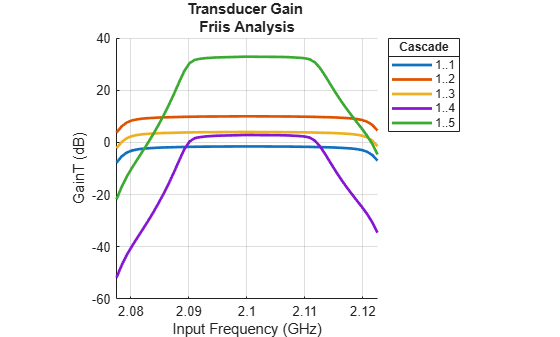Plot S-parameters of an RF system on a Smith Chart and a Polar plot.

s = smithplot(b,1,1,'GridType','ZY');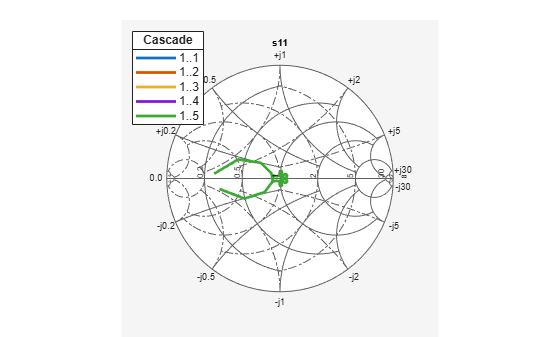p = polar(b,2,1);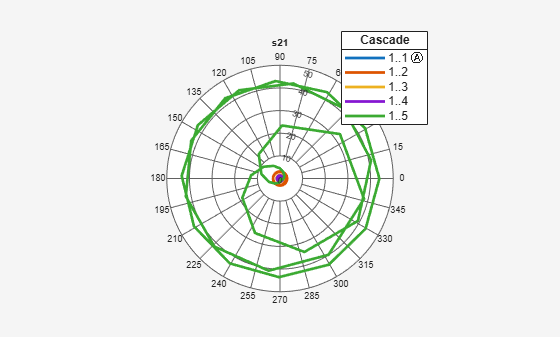Create two modulators, m1 and m2, with output-referred second-order intercept set to 20 and available power gain set to 3.

m = modulator('Gain',3,'OIP2',20,'ImageReject',false,'ChannelSelect',false);
m2 = modulator('Gain',3,'OIP2',20,'ImageReject',false,'ChannelSelect',false);

Create a RF budget object specifying the input frequency of the signal, power applied at cascade, and signal bandwidth. Select HarmonicBalance as solver method to compute nonlinear effects such as IIP2 and OIP2.

b = rfbudget([m m2],2.1e9,-30,100e6,'Solver','HarmonicBalance')
b =
rfbudget with properties:

Elements: [1x2 modulator]
InputFrequency: 2.1 GHz
AvailableInputPower: -30 dBm
SignalBandwidth: 100 MHz
Solver: HarmonicBalance
WaitBar: true
AutoUpdate: true

Analysis Results
OutputFrequency: (GHz) [  3.1    4.1]
OutputPower: (dBm) [  -27    -24]
TransducerGain: (dB)  [    3      6]
NF: (dB)  [ 3.01  7.783]
IIP2: (dBm) [   17  4.457]
OIP2: (dBm) [   20  10.46]
IIP3: (dBm) [  Inf    Inf]
OIP3: (dBm) [  Inf    Inf]
SNR: (dB)  [60.96  56.19]

Create an amplifier with a gain of 4 dB.

a = amplifier('Gain',4);

Create a modulator with an OIP3 of 13 dBm.

m = modulator('OIP3',13);

Create an nport using passive.s2p.

n = nport('passive.s2p');

Create an rf element with a gain of 10 dB.

r = rfelement('Gain',10);

Calculate the rf budget of a series of rf elements at an input frequency of 2.1 GHz, an available input power of -30 dB, and a bandwidth of 10 MHz.

b = rfbudget([a m r n],2.1e9,-30,10e6);

Show the analysis in the RF Budget Analyzer app.

rfplot(b)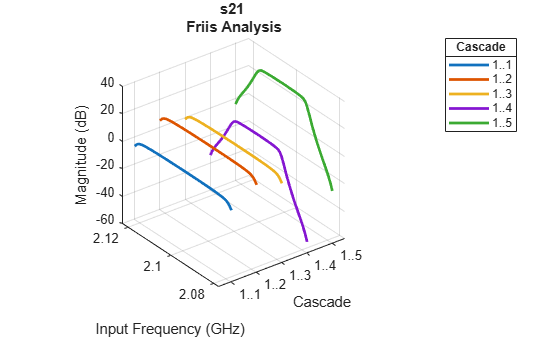Group Delay

To plot the group delay, first plot the S11 data for the RF System.

rfplot(b,1,1)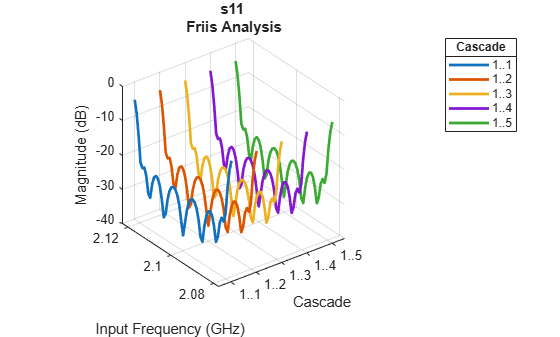Use the Group Delay option on the plot graph to plot the group delay of the RF system.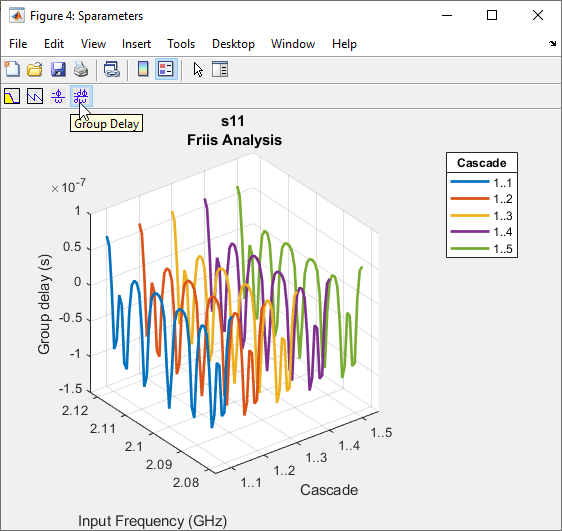Phase Delay

Use the Phase Delay option on the plot graph to plot the phase delay of the RF System.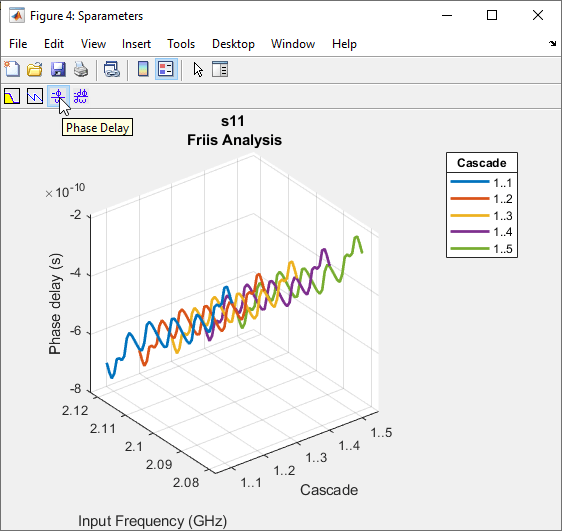Tips

• The Touchstone file in the nport object must be passive at all specified frequencies. To make n-port S-parameters passive, use the makepassive function.

Algorithms

expand all

ABCD parameters are used in the computation of S-parameters of the cascade for Friis Solver. When S21 = 0, conversion to ABCD results in NaNs. For such cases, modifications to the S-parameters are made as follows: